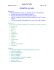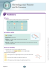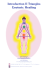# An example on five classical centres of a right angled triangle, pdf

## Transcription

An example on five classical centres of a right angled triangle, pdf
```An example on five classical centres of a right angled triangle
Yue Kwok Choy
Given O(0, 0), A(12, 0), B(0, 5) .
The aim of this small article is to find the
co-ordinates of the five classical centres of the
∆ OAB and other related points of interest.
We choose a right-angled
angled triangle for
simplicity.
(1) Orthocenter:
The three altitudes of a triangle meet in
one point called the orthocenter.
(Altitudes are perpendicular lines from
vertices to the opposite sides of the
triangles.)
If the triangle is obtuse, the orthocenter is outside the triangle. If it is a right triangle,
the orthocenter is the vertex which is the right angle.
Conclusion : Simple, the orthocenter = O(0, 0) .
(2) Circum-center:
The three perpendicular bisectors of the sides of
a triangle meet in one point called the
circumcenter. It is the center of the circumcircle,
the circle circumscribed about the triangle.
If the triangle is obtuse, then the circumcenter is
outside the triangle. If it is a right triangle, then
the circumcenter is the midpoint of the
hypotenuse. (By the theorem of angle in
semi-circle
circle as in the diagram.)
Conclusion : the circum
circum-centre, E = ,
6,2.5
1
Exercise 1:
\$ 2.5 6.5 .
circum-circle above is given by: x \$ 6 y
(a) Check that the circum
(b) Show that the
he area of the triangle with sides a, b, c and angles A, B, C is
2R sin A sin B sin C ,
where R is the radius of the circum-circle
circum
.
(3) Centroid:
The three medians (the lines drawn
from the vertices to the bisectors of
the opposite sides) meet in the
centroid or center of mass. The
centroid divides each median in a
ratio of 2 : 1.
Since OR : RA = 1 : 1, we have R = 6, 0.
Since BC : CR = 2 : 1, we therefore have
"(" "".
Centroid, C = Conclusion :
,
4, * .
A x , y , B x , y , C x* , y* , then the
Exercise 2: Prove that iif
,- ,. ,
,/ 0- 0. 0/
coordinates of the centroid of ∆ABC is given by *
,
*
.
(4) In-center: The three angle
bisectors of a triangle meet in one
point called the in-center.
center. It is the
center of the in-circle,
circle, the circle
inscribed in the triangle.
Let OA = a = 12,
OB = b = 5
AB = c = √12 5 13
Semi-perimeter, s =
*
15
The radius of the incircle = r = EX = EY = EZ
Then Area of ∆OAB
OAB = Area of ∆EOA + Area of ∆EOB+
EOB+ Area of ∆EAB
∴ r !
"
"
r sr
2
2
Conclusion :
In-centre
centre : E = (r, r) = (2, 2)
In-circle
circle : x \$ 2 y \$ 2 2
Exercise 3:
Prove that the radius of a general ∆ABC (not just right) with sides a, b, c and
r6
semi-perimeter
perimeter s is given by :
!5
!5
!5
!
(5) Ex-center: An ex-circle of the triangle is a
circle lying outside the triangle, tangent to
one of its sides and tangent to the
extensions of the other two.
Every triangle has three distinct ex
ex-circles,
each tangent to one of the triangle's sides.
The center of an ex-circle
circle is tthe intersection
of the internal bisector of one angle and the
external bisectors of the other two.
As in the diagram,
Area of ∆OAB
= Area of ∆E1AB + ∆E1OB - ∆E1OA
ab cr br ar
bc\$a
a b c \$ 2a
\$
7
8 r 7
8 r
2
2
2
2
2
2
4
=
Similarly, r ∴ r Conclusion :
!5
\$ a9 r s \$ ar
"
5
!5
=
=
10
"
5
3, r* !5
=
"
5*
Ex-centre
centres : E r , \$r 10, \$10
E \$r , r \$3,3
E* r* , r* 15,15
15
Exercise 4:
(a) Write down the equations of the ex
ex-circles in the above.
(b) Prove that for a general triangle (not just right) the radii of the ex-circles
ex
are
r 6
!
!5
!5
!5
,rr 6
!
!5
!5
!5
,r* 6
!
!5
!5
!5
3
```

### Pythagoras’ Theorem Now have a go yourself .. . .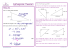### MA.7.A.1.3: Scale Factor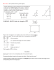### Incenter vs Circumcenter Triangle Worksheet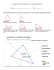### See details. - Math Awareness Month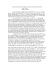### Practice 1.3 KEY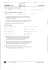### Triangle Play Balls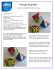### wizBRAIN - W4Kangoeroe### HSM12CC_GM_05_03_CM - Newark Catholic High School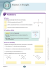### July 11 Newsletter - PCEA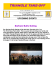### puzzler - Brain Quest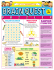### square, circle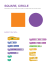### Registration Form - NC-CCIM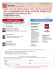### Report (PDF 1.36MB) - European Go Federation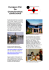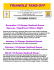### HSM12CC_GM_05_04_CM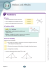###    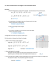### Teacher: Ms. Williams Name: ____________________________________ 1.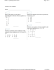### Nepal Engineering College Changunarayan, Bhaktapur Compiled by: Asst. Prof. Daya Ram Budhathoki dayaramb(AT)nec.edu.np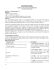### Course 3 Unit 3 Practice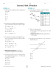### Geometry Notes PERIMETER AND AREA